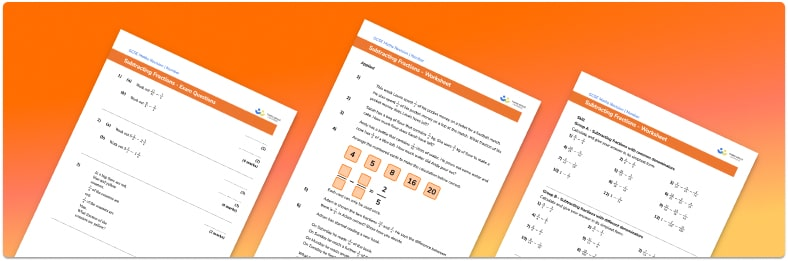# Subtracting Fractions Worksheet• Section 1 of the subtracting fractions worksheet contains 20+ skills-based subtracting fractions questions, in 3 groups to support differentiation
• Section 2 contains 3 applied subtracting fractions questions with a mix of word problems and deeper problem solving questions
• Section 3 contains 3 foundation and higher level GCSE exam style subtracting fractions problems
• Answers and a mark scheme for all subtracting fractions questions are provided
• Questions follow variation theory with plenty of opportunities for students to work independently at their own level
• All questions created by fully qualified expert secondary maths teachers
• Suitable for GCSE maths revision for AQA, OCR and Edexcel exam boards

• This field is for validation purposes and should be left unchanged.

You can unsubscribe at any time (each email we send will contain an easy way to unsubscribe). To find out more about how we use your data, see our privacy policy.

### Subtracting fractions at a glance

We can subtract fractions using different methods. In order to subtract simple fractions with the same denominator, we just subtract the numerators and leave the denominator the same. For example, frac{3}{5} - frac{1}{5} = frac{2}{5} . If two fractions have different denominators, we need to rewrite them as equivalent fractions with a common denominator before we can subtract them.

To write fractions with a common denominator, the first step is to find a common multiple of the denominators. This could be the least common multiple or any other common multiple. We then need to find equivalent fractions to the originals with denominators equal to the common multiple. Once we have two fractions with like denominators, we can subtract the numerators, leaving the denominators the same.

Mixed numbers can be subtracted in the same way as proper fractions. It is often easiest to write the mixed number as an improper fraction before following the same process as above. Once the fractions have been subtracted, any improper fraction can be written back as a whole number and a fraction.

Looking forward, students can then progress to additional adding and subtracting fractions worksheets and other number worksheets, for example a multiplying and dividing decimals worksheet or an order of operations worksheet.For more teaching and learning support on Number our GCSE maths lessons provide step by step support for all GCSE maths concepts.

## Do you have KS4 students who need more focused attention to succeed at GCSE?There will be students in your class who require individual attention to help them succeed in their maths GCSEs. In a class of 30, it’s not always easy to provide.

Help your students feel confident with exam-style questions and the strategies they’ll need to answer them correctly with our dedicated GCSE maths revision programme.

Lessons are selected to provide support where each student needs it most, and specially-trained GCSE maths tutors adapt the pitch and pace of each lesson. This ensures a personalised revision programme that raises grades and boosts confidence.

Find out more